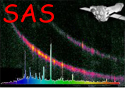XMM-Newton Science Analysis System

rgsbkgmodel (rgsbkgmodel-1.5.1) [xmmsas_20211130_0941-20.0.0]

# Algorithm

``` compute the background lightcurve of the problem event file applying
the following selection expression

CCDNR==9 AND (XDSP_CORR < -3.E-4 OR XDSP_CORR > 3.E-4)

From the lightcurve, calculate the fraction of the total time for
each of the pre-defined levels of background.

Compute the background template spectra by weighting each of the
template spectra by the corresponding factor calculated from the
ligthcurve.
```

XMM-Newton SOC -- 2021-11-30